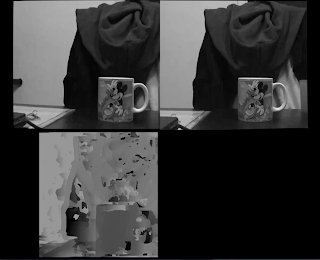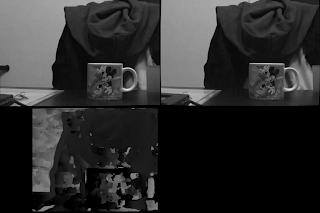## Wednesday, January 1, 2014

### Better Stereo (?) using Rectified Left and Right images and stereo Q matrix with and without zero disparityLeft and right images with zero disparity calibration and the resulting disparity map. With higher possible disparity values (this is a paremeter for the alogithm), the left of the map is black, but gives better results in general for the found disparity.
So the stereo camera is important to provide 3D information of the world that is currently visible. The difference in position of an object in the left and right images defines the objects position. With this information we can start reconstructing the world around the camera. So there are two points:
1.) Determining the relative position of objects in the left and images in terms of how many pixels the object's position is different from left to right. This is done using something like stereo block matching.
2.) Translate this pixel difference in to real world values like centimeters and meters using the camera parameters.

Before 1 and 2 can be done, the left and right images need to be rectified to fit into the normalized camera reference point, so the algorithms can correctly run. See references on epipolar lines or rectified stereo images.

The one issue I ran into was how was the Q matrix in 2 above defined. ScaViSLAM uses a Q matrix with "zero disparity" this means that the Q matrix looks like this (see StereoCamera::Q()):
1    0    0    Cx
0    1    0    Cy
0    0    0     f
0    0    1/b  0

where (Cx, Cy) is the camera center point, f is the camera focal distance and b is the baseline distance (distance between the left and right camera center points). To obtain this value using openCV function cvStereoRectify, you must use the CV_CALIB_ZERO_DISPARITY flag. If you don't use this then your matrix looks like:

1    0    0    Cx
0    1    0    Cy
0    0    0     f
0    0    1/b  b'/b

Where b' is the shift between the 2 cameras. Using a non zero disparity should help to conserve pixel area after rectification. But essentially both should work, its just a question of what the different algorithms are expecting. ScaViSLAM uses a hierarchy of images, so recalculates the camera parameters for each scale, which it then needs the zero disparity matrix. You can do the same calculations for non-zero disparity, but it was easiest to get started using the CV_CALIB_ZERO_DISPARITY flag.Left and right images with non-zero disparity calibration and the resulting disparity map. More area of the captured images is preserved. The disparity map looks quite difference due to the different Q matrix that will reproject the points

I cam across this blog: http://robotpingpong.blogspot.de/2013/03/small-progress-in-triangulation.html. Here the results for the rectification are much more warped. I guess because his 2 PS3 cameras are not so closely arranged. The raspberry pi camera gives good results here because you can mount then closely (6.5cm apart). His cameras I think were 50cm apart.